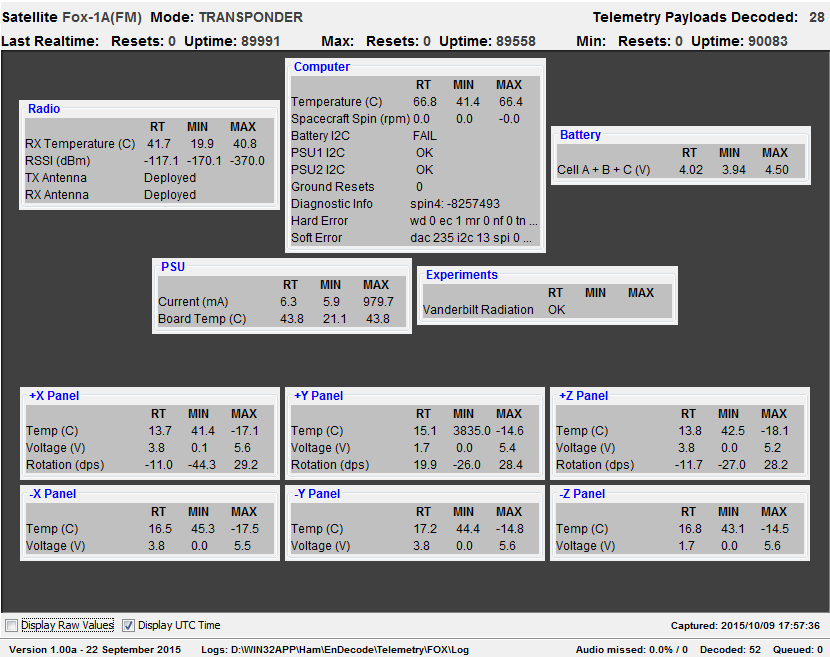# FOX-1A Telemetry 09-10-2015

FOX-1A Telemetry 09-10-2015

```Fox-1a Telemetry

96 bytes of telemetry was received on  Fri, 09 Oct 2015 17:56:03 UTC

Fox ID=1, reset count=0, uptime=89991, type=1
Rx Antenna Deploy=1, Tx Antenna Deploy=1,Telemetry Reset Count=0
Vulcan Communications OK
Battery I2C Telem Failure=1, PSU1 I2C Telem Failure=0, PSU2 I2C Telem Failure=0

Converted values:

Battery Voltage= 4.0, IHU Junction Temp=66.8C
+X Panel Volts= 3.8, +Y Panel Volts= 1.7, +Z Panel Volts= 3.8
-X Panel Volts= 3.8, -Y Panel Volts= 3.8, -Z Panel Volts= 1.7
+X Panel Temp=13.7C, +Y Panel Temp=15.1C, +Z Panel Temp=13.8C, PSU Current= 0.0 ma
-X Panel Temp=16.5C, -Y Panel Temp=17.2C, -Z Panel Temp=16.8C, PSU Temp=43.8C
X MEMS=-11.0 dps, Y MEMS=19.9 dps,Z MEMS=-11.7 dps)